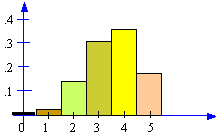### CFA Practice Question

There are 985 practice questions for this topic.

### CFA Practice Question

For the bar graph of random variable x shown below:A. mean = 1.2
B. P(x > 0) = 0
C. P(x <= 5) = 1
D. expected value of x is 1

The sum of all the P(x)s is 1 for any probability distribution. Now, P(x) <= 5 is the sum of all the P(x)s. So, P(x <= 5) = 1.

User Comment
Yooo P(x)< or = 5 is 1. The P(x)< 5 is approx. .82
mtcfa This histogram is somewhat misleading, as per the last section the highest value would be 5.5.
hoiletab That's what i was led to believe initially but really and truly none of the other answers can be logically considered. I think this is a case of choosing the best suited answer is you have a problem interpreting the data. agree?
adenisov Well, that's the point how to understand the picture. I saw it as x is integer, not real, so the is only 6 possible outcomes. Like a dice with different weights on sides.
surjoy Thats not how I interpreted.
P(x=50) = .2
P(x=4) = .35
P(x=3) = .3
p(x=2) = .15
p(x=1) = 0
johntan1979 Not perfect but C is the best possible answer. All other options are way off.
khalifa92 all probabilities are x<=5